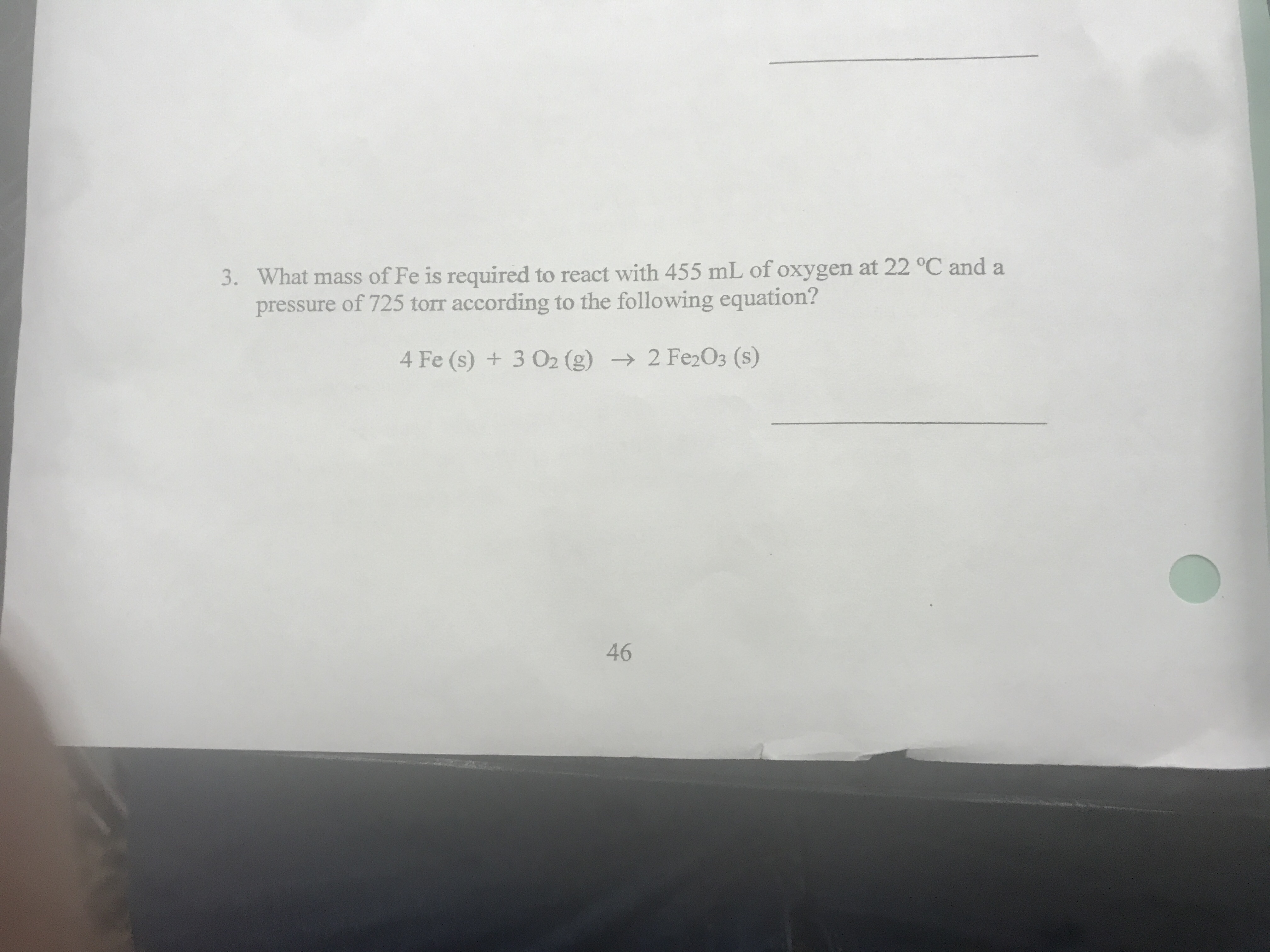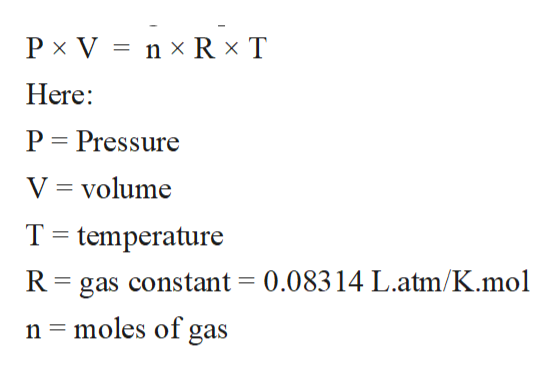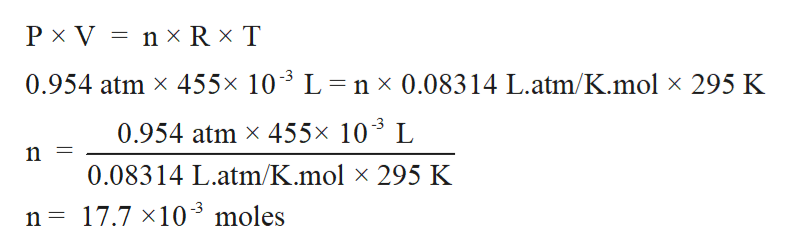# 3. What mass of Fe is required to react with 455 mL of oxygen at 22 °C and apressure of 725 torr according to the following equation?4 Fe (s) +3 O2 (g) 2 Fe2O3 (s)46

Question
6 viewshelp_outlineImage Transcriptionclose3. What mass of Fe is required to react with 455 mL of oxygen at 22 °C and a pressure of 725 torr according to the following equation? 4 Fe (s) +3 O2 (g) 2 Fe2O3 (s) 46 fullscreen
check_circle

Step 1

The gaseous state is the state of matter with least intermolecular force of attraction between molecules and highest kinetic energy. The standard properties of gases like Pressure, volume, and temperature can be calculated with the help of ideal gas equation.

The ideal gas equation can be written as:help_outlineImage TranscriptioncloseP x V n x R x T Here P Pressure V volume T temperature R gas constant = 0.08314 L.atm/K.mol = moles of gas fullscreen
Step 2

Pressure = 725 torr = 0.954 atm (1 atm = 760 torr)

Volume = 455 mL = 455 x 10-3 L

Temperature = 22C = 22 +273 = 295 K

Calculate number of moles of oxygen gas with the help of Ideal gas equation:help_outlineImage TranscriptioncloseP x V n x Rx T 0.954 atm x 455x 103 L nx 0.08314 L.atm/K.mol x 295 K 0.954 atm x 455x 103 L 0.08314 L.atm/K.mol x 295 K 17.7 x103 moles n fullscreen
Step 3

According to balance chemical equation:

4 mole...

### Want to see the full answer?

See Solution

#### Want to see this answer and more?

Solutions are written by subject experts who are available 24/7. Questions are typically answered within 1 hour.*

See Solution
*Response times may vary by subject and question.
Tagged in

### Other